## The energy of a particle.

The physicists of the eighteenth and nineteenth centuries developed the study of mechanics based on Newton's laws and defined the concept of mechanical energy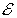of a particle (point-like body with inertial mass m).

In particular, they determined that, if the particle is placed in a conservative field, for every point P in this field we can define a scalar function of the position r of P, denoted by V(r) said potential and that the total energyof this particle in position r and with velocity v is given by the sum of two functions said, respectively,

• kinetic energy K, depending on inertial mass m and on velocity v;
• potential energy U, depending on the potential V(r) and on a quantity q, which we can generically call its charge and which expresses the degree of sensitivity of the particle to the presence of the field. q can be, depending on the field, the gravitational charge, usually called gravitational mass, or the electrical or nuclear charge.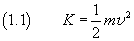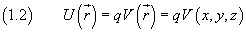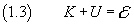Introducing the quantity p, said momentum of the particle, defined as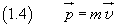we can rewrite the equation (1.1) as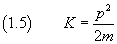and the equation (1.3) as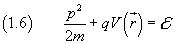From Newton's laws we can deduce that the energyof a particle moving in a conservative field is constant.

Since the energy is concentrated in the particle, the displacement of a particle in space implies flow of energy.

For example, the energy possessed by a bullet fired from a firearm flows in space with the motion of the bullet and when the bullet reaches the target and sticks in it, the energy is transferred to the target.

In conclusion we can say that energy can propagate in space with the motion of one or more particles.